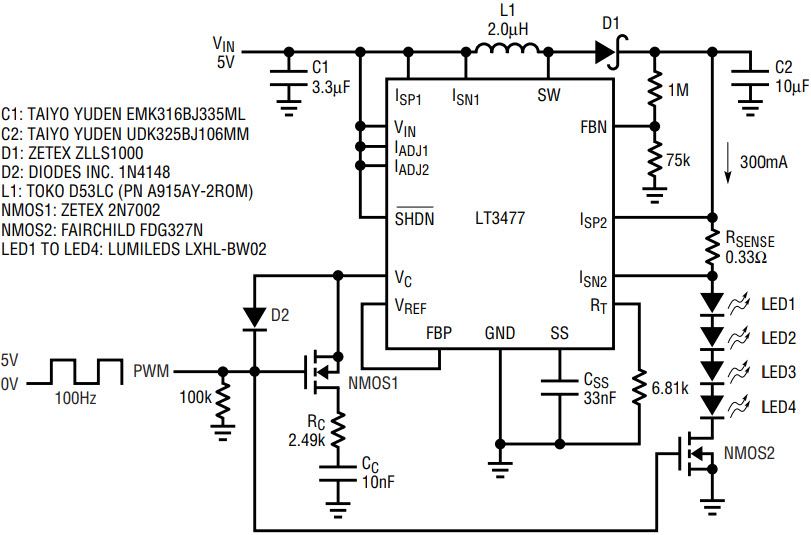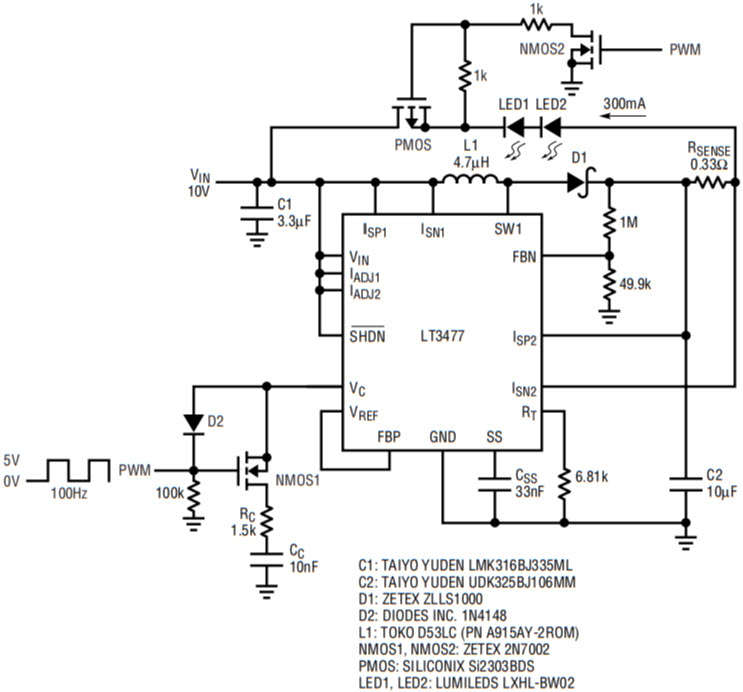# 3A Converter Drives LEDs with 500:1 Dimming

Introduction

For LED applications where a wide dimming range is required, two competing methods are available: analog dimming and PWM dimming. The easiest method is to simply vary the DC current through the LED—analog dimming—but changing LED current also changes its chromaticity (color shift), undesirable in many applications (such as LCD backlights).

The better method is PWM dimming, which switches the LED on and off, using the duty cycle to control the average current. PWM dimming offers several advantages over analog dimming and is the method preferred by LED manufacturers. By modulating the duty cycle of the PWM signal, the average LED current changes proportionally as illustrated in Figure 1. The chromaticity of the LEDs remains unchanged in this scheme since the LED current is either zero or at programmed current. Another advantage of PWM dimming over analog dimming is that a wider dimming range is possible.Figure 1. The average LED current changes proportionally with PWM duty cycle. Many LED manufacturers specify PWM dimming because it offers wider dimming ranges and better LED performance than analog dimming.

The LT3477 is a 3A DC/DC converter that is ideally suited for LED applications. For the LT3477, analog dimming offers a dimming ratio of about 10:1; whereas, PWM dimming with the addition of a few external components results in a wider dimming range of 500:1. The technique requires a PWM logic signal applied to the gate of both NMOS transistors (refer to Figure 2). When the PWM signal is taken high the part runs in normal operation and ILED of 100mV/RSENSE runs through the LEDs. When the PWM input is taken low the LEDs are disconnected and turn off. This unique external circuitry produces a fast rise time for the LED current (see Figure 3), resulting in a wide dimming range of 500:1 at a PWM frequency of 100Hz.Figure 2. A 5V input drives four white LEDs in boost mode with PWM dimming.Figure 3. Rising LED current for the circuit in Figure 2 settles in under 20µs, thus allowing short pulse widths, and high dimming ratios.

The LED current can be controlled by feeding a PWM signal with a broad range of frequencies. Dimming below 80Hz is possible but not desirable due to perceptible flashing of LEDs at lower frequencies. The LED current can be controlled at higher frequencies, but the dimming range decreases with increasing PWM frequency.

In high temperature applications, the leakage of the Schottky diode D1 increases, which in turn, discharges the output capacitor during the PWM “off” time. This results in a smaller effective LED dimming ratio. Consequently, the dimming range decreases to about 200:1 at 85°C.

PWM dimming can be used in boost mode (Figure 2), buck mode (Figure 4) and buck-boost mode (Figure 5). For the typical boost topology, efficiency exceeds 80%. Buck mode can be used to increase the power handling capability for higher current LED applications, A buck-boost LED driver works best in applications where the input voltage fluctuates to higher or lower than the total LED voltage drop.Figure 4. Buck mode converter drives six white LEDs with PWM dimming from a 32V input.Figure 5. Buck-boost mode converter drives two white LEDs with PWM dimming from a 10V input.